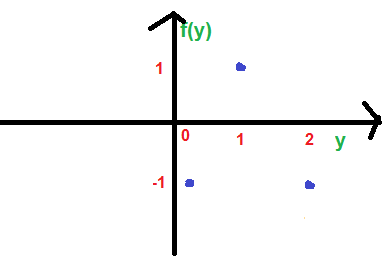# GATE | GATE-CS-2014-(Set-1) | Question 65

• Last Updated : 28 Jun, 2021

A function is continuous in the interval [0, 2]. It is known that f(0) = f(2) = -1 and f(1) = 1. Which one of the following statements must be true.
(A) There exist a y in the interval (0, 1), such that f(y) = f(y+1)
(B) For every y in the interval (0, 1) , f(y) = f(2-y)
(C) The maximum value of the function in the interval (0, 2) is 1
(D) There exist a y in the interval (0, 1), such that f(y) = -f(2-y)

Explanation: